Please wait a minute...
 Chin. Phys. B, 2020, Vol. 29(11): 110303    DOI: 10.1088/1674-1056/abaee8
Special Issue: SPECIAL TOPIC — Quantum computation and quantum simulation
 SPECIAL TOPIC—Quantum computation and quantum simulation Prev   Next

# A two-dimensional quantum walk driven by a single two-side coin

Quan Lin(林泉)1, †, Hao Qin(秦豪)2, † Kun-Kun Wang(王坤坤)1, Lei Xiao(肖磊)1, and Peng Xue(薛鹏)1,, ‡
1 Beijing Computational Science Research Center, Beijing 100084, China
2 Department of Physics, Southeast University, Nanjing 211189, China
 Abstract  We study a two-dimensional quantum walk with only one walker alternatively walking along the horizontal and vertical directions driven by a single two-side coin. We find the analytical expressions of the first two moments of the walker’s position distribution in the long-time limit, which indicates that the variance of the position distribution grows quadratically with walking steps, showing a ballistic spreading typically for quantum walks. Besides, we analyze the correlation by calculating the quantum mutual information and the measurement-induced disturbance respectively as the outcome of the walk in one dimension is correlated to the other with the coin as a bridge. It is shown that the quantum correlation between walker spaces increases gradually with the walking steps. Keywords:  quantum walks      quantum mutual information      measurement-induced disturbance Received:  30 June 2020      Revised:  04 August 2020      Accepted manuscript online:  13 August 2020 Fund: the National Natural Science Foundation of China (Grant Nos. 11674056 and U1930402) and the startup fund from Beijing Computational Science Research Center. KKW acknowledges support from the Project funded by China Postdoctoral Science Foundation (Grant No. 2019M660016). Corresponding Authors:  †These authors contributed equally. ‡Corresponding author. E-mail: gnep.eux@gmail.com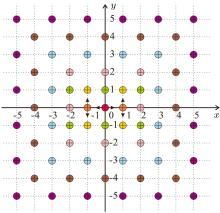Fig. 1.  Schematic for the two-dimensional QW. At the beginning of the walk, the single walker, starts from the position (x0 = 0,y0 = 0) with a two-side quantum coin in his hand. Then the walker flips the coin and takes a step along the x axis to the left or right if the coin turns out to be head or tail. Hence, the walker is possible to appear at positions (−1,0) and (1,0), i.e., the orange points. After this, the walker flips the coin again and the outcome of the coin flipping decides the walker’s movement along the y axis, up or down. Therefore, we may find the walker at positions (−1,−1), (−1,1), (1,−1), and (1,1), i.e., the yellow points in the figure with certain probabilities. We consider a step along the x axis and a step along the y axis respectively of the walker as a single course of our walk. During the second course, the walker possibly gets to the positions of the green points after the first coin flipping and finally arrives at the positions of the pink points and also the initial red point, which are 9 possible positions in total, after the second coin flipping. We omit the intermediate positions the walker may reach from the third course of the walk in the figure. It can be seen that after the n-th course the walk shows a spreading of position distribution including (n + 1)2 possible points on the two-dimensional lattice.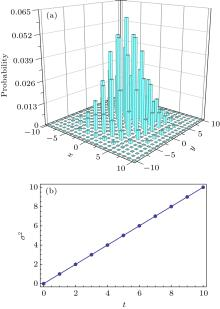Fig. 2.  (a) The position distribution of the two-dimensional CRW after the 10th step. (b) The variance of the position distributions of the two-dimensional CRW for the fist 10 steps.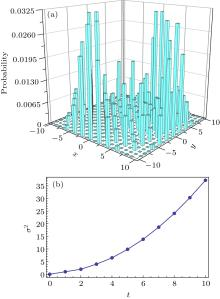Fig. 3.  (a) The position distribution of the two-dimensional QW after the 10th step. (b) The variance of the position distributions of the two-dimensional QW for the fist 10 steps.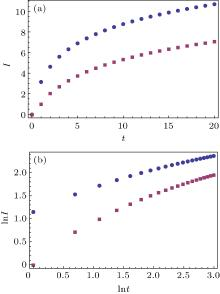Fig. 4.  (a) The quantum mutual information of two-dimensional QW (squares) and of the maximal entangled state (dots). (b) The ln–ln plot of the quantum mutual information of two-dimensional QW (squares) and of the maximal entangled state (dots).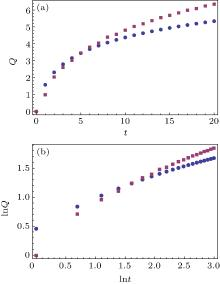Fig. 5.  (a) The measurement-induced disturbance of two-dimensional QW (squares) and of the maximal entangled state (dots). (b) The ln–ln plot of the measurement-induced disturbance of two-dimensional QW (squares) and of the maximal entangled state (dots).